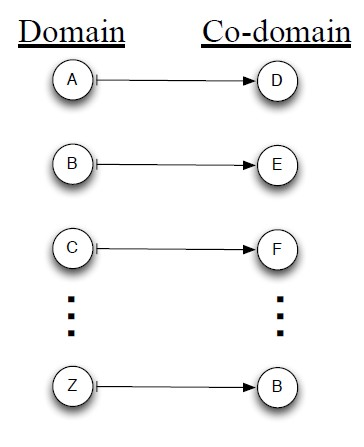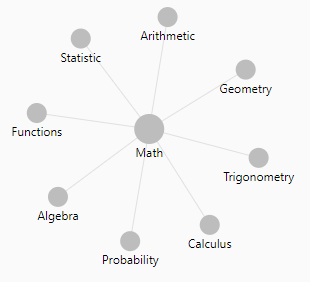# Mathematics - Inverse axiom

• / is inverse of *
• - is inverse of +
• root $\sqrt[n]x$ is the inverse of exponentiation $b^n$

## Linear Algebra

Functions f and g are functional inverses if:

• $f \circ g$ and $g \circ f$ are defined
• and are Function - Identity functions.

Discover More(Function|Operator) - Algebraic (Laws|properties) - Axioms

Property of function and operator Continuous SmoothLinear Algebra - Function (Set)

For each input element in a set , a function assigns a single output element from another set . is called the domain of the function is called the co-domain or in Mathese: The function...Mathematics - Exponential (Euler's number)

is the scientific constant, the exponential. Euler's number The number e is an important mathematical constant that is the base of the natural logarithm. The number e can be defined to be: the unique...Mathematics - Exponentiation (square, cube) - Power

Exponentiation is a binary operation involving two numbers: the base (b) the exponent (n) (or index or power). In text notation or computer language, generally the exponentiation operator...is usually read as b squared. See Quadratus is Latin for square. The inverse function of square is the square root.Number - Root function

The root function is the inverse operation of the exponentiation. With the below exponentiation of the base b to the power of n the base b can be defined back with the root function as: nlogarithm...Tree - Leaf Node

Leaf members/nodes have no children. They are also known as external nodes due to the flower representation where the root is at the center The inverse of a leaf node is a parent node also known as:...Trigonometry - Fourier (Analysis|Synthesis)

Fourier analysis is the study of the way general functions may be represented or approximated by sums of simpler trigonometric functions. In the sciences and engineering,: the process of decomposing...